Picard scheme

A natural generalization of the concept of the Picard variety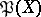for a smooth algebraic varietywithin the framework of the theory of schemes. To define the Picard scheme for an arbitrary-schemeone considers the relative Picard functor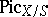in the category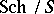of schemes over the scheme. The value of this functor on an-scheme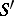is the group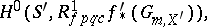whereis the base-change morphism and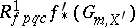is the sheaf in the Grothendieck topology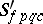of strictly-flat quasi-compact morphisms associated with the pre-sheaf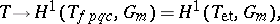and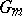denotes the standard multiplicative group sheaf. If the Picard functoris representable on, then the-scheme representing it is called the relative Picard scheme for the-schemeand is denoted by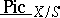. Ifis an algebraic scheme over a certain fieldhaving a rational-point, then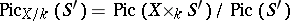for any-scheme. In particular,can be identified with the group of-rational points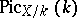of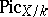if such exists.

If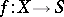is a projective morphism with geometrically-integral fibres, then the schemeexists and is a locally finitely representable separable group-scheme. If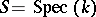, then the connected component of the unit,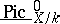, of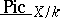is an algebraic-scheme, and the corresponding reduced-schemeis precisely the Picard variety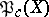. The nilpotent elements in the local rings of the schemegive much additional information on the Picard scheme and enable one to explain various "pathologies" in algebraic geometry over a field of characteristic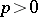. On the other hand, over a field of characteristic 0 the schemeis always reduced . It is also known that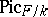is a reduced scheme ifis a smooth algebraic surface and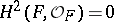.

For any proper flat morphism(finitely representable if the baseis Noetherian) for which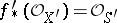, the functoris an algebraic space overfor any base-change morphism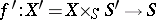. In particular, the functoris representable if the ground schemeis the spectrum of a local Artinian ring.